Hello, fellows, I hope all of you are enjoying your life. In today’s tutorial, we will have a look at Diode Models. A diode is an electronic device that has 2 terminals positive terminals called an anode and a negative terminal called a cathode. Currently, almost every electronic devices use a diode. The main function of a diode is to convert alternating current into DC current. There are such appliances that do not operate on AC supply as we know in our power system AC is generated and used. So in these devices rectifiers circuits are used to convert AC into DC.

If the rectifier converts full wave of ac into dc than it is known as a full-wave rectifier and if convert half wave it called a half-wave rectifier. In today’s post, we will have a detailed look at the different diode models and compare them with the biasing conditions and find their parameters.  In the previous tutorial, I write a complete post on V-I characteristics of a diode. It will help you to understand the modeling of a diode. So let’s get started with Diode Models.

## Diode Models

• For circuit analysis and calculation of different parameters of diodes, mathematic expressions are formed called diode models in electronics.
• As we know V-I curve of a diode is not linear for the understanding of practical behavior of diode simple circuits model of diode needed.
• In today’s post, we will discuss the diode models for ideal and practical diode and compare them with the detailed.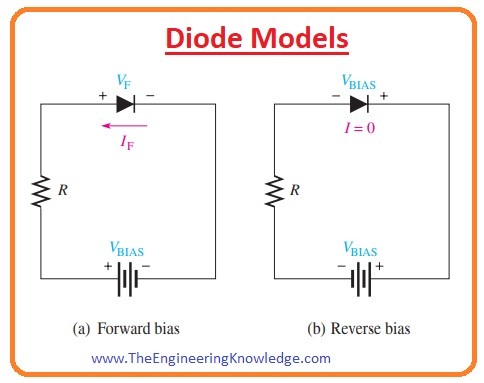## Diode Bias Connections

### Diode Forward-Bias

• In the given figure, the circuit denoted as (a) shown the forward biasing of a diode.• In this circuit, you can see that anode of the diode is connected with the positive terminal of the battery and the negative terminal of the battery with the cathode of the diode.
• The resistance between anode and battery is used to limit the current to save the value.
• (IF) is the forward current flowing through cathode to the anode and VF is the forward voltage drop across the diode is equal to the potential barrier of a diode.

### Diode Reverse-Bias Connection

• In the above figure, the circuit denoted as (b) shows the reverse-biased connection of diode.
• In this circuit positive terminal of a battery is connected to the cathode of diode and the negative terminal is connected with the anode.
• The reverse-biased current is very less and can be neglected and there is no need of limiting resistance in reverse biasing.
• In-circuit you can see that all Vbise or applied voltage is shown across the diode.

## Ideal Diode Model

• In the given figure, the two circuits are shown first discuss the forward biasing ideal diode model and second in reverse biasing ideal diode model.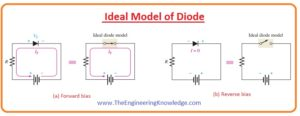• You can see in forward biased condition diode is behave like a closed switch and reveres biased like an open switch.
• In ideal condition the potential barrier, dynamic resistance in forward biasing and reverse current is ignored.
• This model is inadequate for troubleshooting when you are trying to define if the diode is functioning correctly.
• in given Figure V-I characteristics of an ideal diode is shown.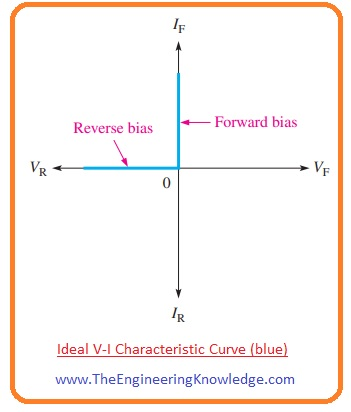• As the dynamic resistance and potential barrier are ignored so the voltage across the diode is zero in forward biasing.
• In forward bias, the curve of an ideal diode is shown on the vertical Y-axis in blue color.

VF =0

• The value of forward current (IF) across the diode can be found by using the value of limiting resistance and biased voltage using Ohm’s Law.

IF =VBIAS /RLIMIT

• As reverses current is ignored in an ideal diode and its value is supposed to be ‘0’. It is shown in the V-I curve on negative axis.

IR=0A

• The value of reverse voltage is equal to the biased voltage.

VR =VBIAS

## Practical Diode Model

• In the given figure there are two circuits shown for practical diode first is denoted as (a) it is forward bias and second denoted as (b) is reverse bias.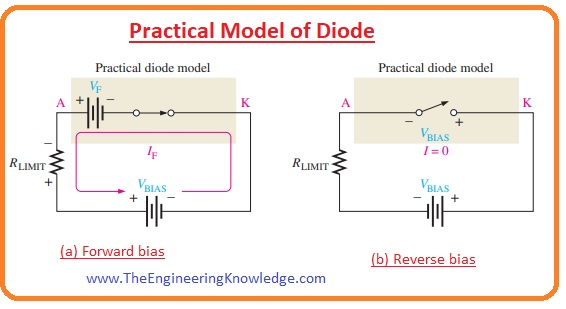• in a practical model of diode potential barrier is included. In a forward-biased circuit, you can see that diode is like a closed switch having a series voltage source (VF) equal to the (0.7) volts its positive side attached with the anode.
• This source is a potential barrier voltage, not a practical voltage source. The value of a biased voltage should be larger to operate the diode.
• While in reverse bias the circuit of a diode is like an open switch and there is no effect in reverse biasing.
• in a given figure, the V-I curve for a practical model of a diode is shown.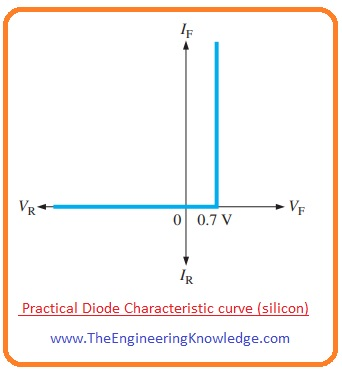• At a positive X-axis of the diode forward biased condition of the diode is shown in this biasing potential barrier is included so it shown in the graph.

VF = 0.7 V

• The forward current is determined as follows by first applying Kirchhoff’s voltage law to Figure 2–16(a):
• If we apply KVL on the forward-biased circuit denoted as (a) then the value of forwarding current is given as.

VBIAS – VF – VRLIMIT = 0

VRLIMIT = IFRLIMIT

• Now we have.

IF= (VBIAS-VF)/ RLIMIT

• As the reverse current is zero as shown on the negative X-axis of the curve.

IR = 0 A

VR = VBIAS

• For troubleshooting of a less voltage circuit’s practical model of a diode is the best option.
• In this situation, 0.7 volts around diode can be beneficial and should be taken into consideration.
• Friends if you have to design a basic circuit of diode than you should prefer a practical diode model.

## Complete Diode Model

• Now we discuss the complete model of the diode that has potential barriers and dynamic forward resistance.
• With these 2 parameters, this complete model also has high-value interior reverse resistance.
• In this model, the reason of reverse resistance inclusion is that this resistance provides a path for the reverse current movement.
• In the given figure, two circuits are shown for a complete model of a diode. The circuit denoted as (a) shows the complete model for forward bias and the circuit denoted as (b) describes the reverse-biased complete model.• In forward bias, the condition diode is like a closed switch having a potential barrier (VB) and less value forward dynamic resistance.
• While in reverse biased condition diode is operating like an open circuit switch and has reverse interior resistance in parallel.
• In reverse biasing potential barrier is not included as it does not have any effect in this biasing.
• The V-I curve for a complete model of a diode is shown in a given figure.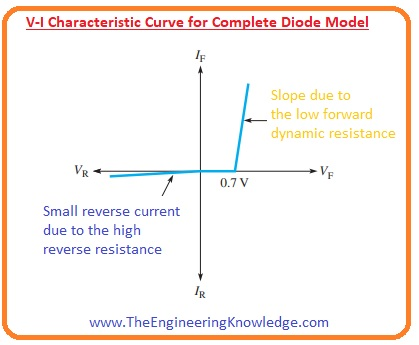• During forward biasing as a potential barrier and dynamic resistance is included so there is (VF) forward voltage across diode exits.
• The voltage (VF) will be equal to the sum of the potential barrier voltage and the voltage drop across dynamic resistance. This voltage is shown on the positive X-axis in the curve.
• The graph in forward bias is like a slope due to an increment in a voltage drop across the dynamic resistance with the increase in current.
• The given below mathematical expression is for a complete model of a silicon diode.

VF = 0.7 V + IFr’d

IF = (VBIAS – 0.7 V)/(RLIMIT + r’d)

• During reverse biasing reverse current IR taken into consideration and shown on the negative X-axis of the curve.
• On this curve breakdown region of the diode is not shown as it does not save the operation region for some diodes.
• Due to the complex calculations, the complete model is not suitable for troubleshooting of diode.
• The complete model can be used when the computer is used to solve complicated calculations.

## Faqs

1. What is a diode model?

A diode model is a mathematical representation of an electrical functioning diode. This model is helpful to find the value of voltage loss of diode curent passing through it.

2. What are the different types of diode models?

The three main types of diode models are

• Ideal diode model: in this model configuration there is no voltage loss in the forward-based state and infinite resistance for reverse bias
• Constant voltage drop model: It defines that the diode comes with constant voltage for forward base state that re 0.7 v for silicon and infinite resistance for reverse biased state
• Shockley diode model: This model is correct than the constant voltage loss model and makes an exponential relation between forward voltage and current

3. Which diode model should I use?

The use of a diode model based on accuracy required and circuit complication. Such as the constant voltage loss model is mostly used. In the case of the circuit where a high level of accuracy is needed, the Schottky model is used

4. How do I use a diode model to analyze a circuit?

These points are used to  analyze a circuit after selecting the diode model

1. Get the diode location in the circuit and mention the curent and voltage for the diode.
2. Curent and voltage can be measured about each diode in the use of a diode model
3. By using these measured curent and voltage we can measure curent and voltage about other circuit components

5. What are some of the limitations of diode models?

Each diode model comes with differnt parameters and features of the diode, So they not be used for any circuit and operating value of the diode. Like Shockley diode model does measure the temperature effect on the diode

7. What are some of the parameters that are used in diode models?

• Forward voltage drop:
• Reverse breakdown voltage: .
• Saturation current:
• Dynamic resistance:

8. How can I measure the parameters of a diode?

curve tracer or a semiconductor parameter analyzer used for the measurement of diode parameters

9. How can I model a diode in a circuit simulator?

For modeling the diode in the circuit simulator get teh proper diode model from the simulator’s library and connect it to the circuit diagram

10. What are some of the common mistakes that people make when modeling diodes?

• Wrong diode model for circuit analyses
• Temperature effect not considered
•  dynamic resistance of the diode not taken

11. How can I avoid making mistakes when modeling diodes?

• The use of proper diode model.
• Measuring the temperature value of the circuit.
•  dynamic resistance of the diode

13. What are some of the applications of diode modeling?

• Circuit design
• Device characterization
• Process control
• Fault diagnosis

What is ideal model of diode?

The ideal diode model is the simplest model of a diode, and it defines that the diode is an accurate switch that can be close and opened easily. When the diode is in the forward-biased state it is close and there is zero value of resistance. If the diode in a reverse biased state it is pen and provdies infinite resistance.

What are the 5 parameter diode models?

• Forward voltage:
• Reverse breakdown voltage:
• Saturation current:
• Diode resistance:
• Diode capacitance

What is 4 diodes?

• Full-wave bridge rectifier: converts AC voltage to DC voltage.
• Voltage doubler: It doubles the voltage of an input source
• Voltage clipper: It restricts the voltage of an input signal to a defined value.

What are the 4 characteristics of a diode?

The 4 main characteristics of a diode are:

• Conduction:
• Forward voltage:
• Reverse breakdown voltage:
• Diode resistance:

What is diode and classification?

A diode is a two-terminal semiconductor device that allows current in one direction, but not in the reverse direction. Diodes have different types based on their construction materials and characteristics. Some main types are

• Semiconductor diodes: It is a commonly used type of diode silicon and germanium is used to make these diode
• Light-emitting diodes (LEDs): In the forward-biased state it releases light
• Photodiodes: When light collide with this diode it causes current
• Varactor diodes: These diodes are used for reliable capacitance
• Zener diodes: They offer constant voltage loss when they are reverse-biased.

What is the most popular type of diode?

semiconductor diode is a very simple and commonly used diodeThey are less price and easy to make

Which diode is the fastest?

Schottky diode is a high-speed diode. It has low forward voltage loss and a small reverse recovery time. So they are used in RF circuits and high-speed switching power supply

What is the unit of diode?

There is no certain unit of a diode. diodes are defined on the base of their forward, reverse biased voltages

Which diode model is the most accurate?

The exponential diode model is the correct model of a diode. It over all electrical characteristics of the diode, like the forward voltage loss, saturation current, reverse breakdown voltage,  diode resistance, and diode capacitance.

What is the difference between ideal and practical diodes?

An ideal diode is an accurate switch that is either open or closed. A practical diode is not a switch, and it has some resistance and capacitance. So practical model will operate curent in a single way and block in other direction

What are the applications of a diode?

• Power supplies: transform AC voltage to DC voltage.
• Amplifiers: They are used to amplify signals.
• Signal processing circuits: They are used for filter signals and to do signal-processing tasks.
• Displays: displays like LED displays and LCD displays are with the use of diode

What is the size of a diode?

Diode sizes are made according to their power rating and application. Small signal diodes can be as small as a few millimeters in diameter, while power diodes can be many centimeters in diameter.

What are the two elements of a diode?

the anode and the cathode are the main elements of the diode. The anode is the positive pin of the diode, and the cathode is the negative

What is diode value?

forward voltage loss of the diode is the diode value that must be given to the diode in order to turn it on.

Are diodes AC or DC?

Diodes can be employed in both AC and DC circuits. But mostly used in DC circuits

Why is it called a diode?

The  “diode” get from Greek words “di” (meaning “two”) and “hodos” (meaning “path”). Since they have two pins

What is the symbol of a diode?

The symbol of a diode is a triangle with a bar at the end point. The bar defines the cathode of the diode.

So friends that is the detailed post on the diode models if you have any queries about this post ask in the comments. See you in the next interesting tutorial. Thanks for reading. Have a good day.

1. ds18b20 temperature sensor says:

Hi to all, how is the whole thing, I think every one is getting more from this web page,
and your views are pleasant in support of new people.

2. vr301 potentiometer says:

3. fredrix blue label ultrasmooth canvas says:

My brother suggested I might like this web site. He was entirely right.
This post truly made my day. You cann’t imagine just how much time I had spent
for this information! Thanks!

4. Shakia Brinegar says:

I know this if off topic but I’m looking into starting my own blog and was curious what all is needed to get set up? I’m assuming having a blog like yours would cost a pretty penny? I’m not very internet savvy so I’m not 100 certain. Any tips or advice would be greatly appreciated. Thank you

5. Christine says:

Hello, i’m an EEE student currently studying semiconductor.
Are the currents provided in the model-diagrams above based on “electron’s direction” as opposed to “conventional current direction”?

I’m fairly new to the concept of electricals, it’d be really helpful if you could clarify this for me.